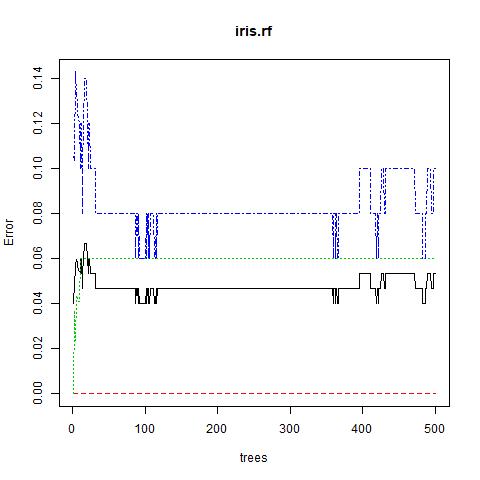Related Articles

# Random Forest Approach for Classification in R Programming

• Last Updated : 08 Jul, 2020

Random forest approach is supervised nonlinear classification and regression algorithm. Classification is a process of classifying a group of datasets in categories or classes. As random forest approach can use classification or regression techniques depending upon the user and target or categories needed. A random forest is a collection of decision trees that specifies the categories with much higher probability. Random forest approach is used over decision trees approach as decision trees lack accuracy and decision trees also show low accuracy during the testing phase due to the process called over-fitting. In R programming, `randomForest()` function of `randomForest` package is used to create and analyze the random forest. In this article, let’s discuss the random forest, learn the syntax and implementation of a random forest approach for classification in R programming, and further graph will be plotted for inference.

#### Random Forest

Random forest is a machine learning algorithm that uses a collection of decision trees providing more flexibility, accuracy, and ease of access in the output. This algorithm dominates over decision trees algorithm as decision trees provide poor accuracy as compared to the random forest algorithm. In simple words, the random forest approach increases the performance of decision trees. It is one of the best algorithm as it can use both classification and regression techniques. Being a supervised learning algorithm, random forest uses the bagging method in decision trees and as a result, increases the accuracy of the learning model.

Random forest searches for the best feature from a random subset of features providing more randomness to the model and results in a better and accurate model. Let us learn about the random forest approach with an example. Suppose a man named Bob wants to buy a T-shirt from a store. The salesman asks him first about his favourite colour. This constitutes a decision tree based on colour feature. Further, the salesman asks more about the T-shirt like size, type of fabric, type of collar and many more. More criteria of selecting a T-shirt will make more decision trees in machine learning. Together all the decision trees will constitute to random forest approach of selecting a T-shirt based on many features that Bob would like to buy from the store.

#### Classification

Classification is a supervised learning approach in which data is classified on the basis of the features provided. As in the above example, data is being classified in different parameters using random forest. It helps in creating more and meaningful observations or classifications. In simple words, classification is a way of categorizing the structured or unstructured data into some categories or classes. There are 8 major classification algorithms:

Some real world classification examples are a mail can be specified either spam or non-spam, wastes can be specified as paper waste, plastic waste, organic waste or electronic waste, a disease can be determined on many symptoms, sentiment analysis, determining gender using facial expressions, etc.

#### Implementing Random Forest Approach for Classification

Syntax:
randomForest(formula, data)

Parameters:
formula: represents formula describing the model to be fitted
data: represents data frame containing the variables in the model

Example:
In this example, let’s use supervised learning on iris dataset to classify the species of iris plant based on the parameters passed in the function.
Step 1: Installing the required library

 `# Install the required``# Package for function``install.packages``(``"randomForest"``)`

 `# Load the library``library``(randomForest)`

Step 3: Using iris dataset in `randomForest()` function

 `# Create random forest``# For classification``iris.rf <- ``randomForest``(Species ~ ., ``                        ``data = iris, ``                        ``importance = ``TRUE``,``                        ``proximity = ``TRUE``)`

Step 4: Print the classification model built in above step

 `# Print classification model``print``(iris.rf)`

Output:

```Call:
randomForest(formula = Species ~ ., data = iris, importance = TRUE,      proximity = TRUE)
Type of random forest: classification
Number of trees: 500
No. of variables tried at each split: 2

OOB estimate of  error rate: 5.33%
Confusion matrix:
setosa versicolor virginica class.error
setosa         50          0         0        0.00
versicolor      0         47         3        0.06
virginica       0          5        45        0.10
```

Step 5: Plotting the graph between error and number of trees

 `# Output to be present``# As PNG file ``png``(file = ``"randomForestClassification.png"``)``  ` `# Plot the error vs ``# The number of trees graph``plot``(iris.rf)``  ` `# Saving the file``dev.off``()`

Output:Explanation:
After executing the above code, the output is produced that shows the number of decision trees developed using the classification model for random forest algorithms, i.e. 500 decision trees. The confusion matrix is also known as the error matrix that shows the visualization of the performance of the classification model.

My Personal Notes arrow_drop_up